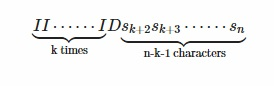# MAXMATCH - Editorial

MEDIUM

### PREREQUISITES:

Combinatorics, Dynamic Programming

### PROBLEM:

Given a bipartite graph having partites X and Y. Partite X will contain all permutations of Set S = {1,2,…n}. Partite Y will contain all strings of length (N-1) having characters ‘I’ and ‘D’ only.There will be an edge between permutation x and string y if string y is the signature of the permutation x and weight of the edge will be the square of the inversion count in permutation x.
We have to find the maximum weighted matching in the given bipartite graph.

### EXPLANATION:

We are given a bipartite graph in which partite X contains permutations of S and partite Y contains strings of length N-1 containing I and D only. There is an edge between x\in X and y \in Y if and only if y is the signature ( explained in question ) of x. Notice here that degree of all the vertices in partite X is 1 and the degree of a vertex in partite Y will be equal to the number of permutations that satisfy this particular signature string corresponding to that vertex. Hence to get the maximum matching, for each vertex in partite Y, we have to select the edge with maximum weight. Now the question arises that which permutation will give the maximum inversion count corresponding to a particular signature string.

It is not very difficult to note that the lexicographically greatest permutation will give us the maximum inversion count. Lets digress a little bit, assume that the weight of the edges is equal to the inversion count of the permutation instead of square of it. In this case, a naive solution is very easy i.e. for each signature string find the inversion count of lexicographically greatest permutation and add it to the answer. But by dynamic programming we can reduce the time complexity of the algorithm.

Define f(n) as the sum of inversion count of lexicographically greatest permutation of all signature strings of length n. Consider a particular class of signature strings of length n in which exactly first k characters are I. A general string of this class will look like thisThe lexicographically greatest permutation will have n-k+1, n-k+2 … n+1 as its first k+1 number ( solve this problem if you are unsure about constructing lexicographically greatest permutation of a given signature string ). So due to this part number of inversion count will be (k+1)*(n-k) and there are 2^{n-k-1} strings which has exactly first k characters as I. So the inversion count due to this class of stings will be (k+1)(n-k)2^{n-k-1} + f(n-k-1). Hence our dp formulation will be as follows

f(n) = \sum_{k=0}^{n-1}(k+1)(n-k)2^{n-k-1} + f(n-k-1)

Coming back to our original problem where we have the square of the inversion count as the edge weight. The solution to this problem is just a extension of what we have solved above. Define g(n) as the sum of square of inversion count of lexicographically greatest permutation of all signature strings of length n. Consider the class of signature strings in which exactly first k characters are I. The answer for this class of strings will be (k+1)^2(n-k)^22^{n-k-1} + 2(k+1)(n-k)f(n-k-1)+g(n-k-1). Hence our dp formulation will be as follows

g(n) = \sum_{k=0}^{n-1}(k+1)^2(n-k)^22^{n-k-1} + 2(k+1)(n-k)f(n-k-1)+g(n-k-1)

Now precompute all the f(n) and g(n), then answer each test case in \mathcal{O}(1) time. The time complexity of the algorithm is \mathcal{O}(n^2).

### AUTHOR’S AND TESTER’S SOLUTIONS:

Author’s solution can be found here
Tester’s solution can be found here

3 Likes

How did you get the second term in equation of g(n)?

Please explain how did you derive the formula for g(n)?

please explain second folmula in little breif